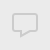## 量化分析

#### numpy indices的用法

Suppose you have a matrix M whose (i,j)-th element equals

M_ij = 2*i + 3*j
One way to define this matrix would be

i, j = np.indices((2,3))
M = 2*i + 3*j
which yields

array([[0, 3, 6],
[2, 5, 8]])
In other words, np.indices returns arrays which can be used as indices. The elements in i indicate the row index:

In : i
Out:
array([[0, 0, 0],
[1, 1, 1]])
The elements in j indicate the column index:

In : j
Out:
array([[0, 1, 2],
[0, 1, 2]])

np.indices((2,3))

img = np.zeros((400,400))
ir,ic = np.indices(img.shape)
circle = (ir-135)**2+(ic-150)**2 < 30**2 # 半径30，圆心在135,150
img[circle]=1

img现在就是一个圆啦
查看全部
```Suppose you have a matrix M whose (i,j)-th element equals

M_ij = 2*i + 3*j
One way to define this matrix would be

i, j = np.indices((2,3))
M = 2*i + 3*j
which yields

array([[0, 3, 6],
[2, 5, 8]])
In other words, np.indices returns arrays which can be used as indices. The elements in i indicate the row index:

In : i
Out:
array([[0, 0, 0],
[1, 1, 1]])
The elements in j indicate the column index:

In : j
Out:
array([[0, 1, 2],
[0, 1, 2]])```

np.indices((2,3))

img = np.zeros((400,400))
ir,ic = np.indices(img.shape)
circle = (ir-135)**2+(ic-150)**2 < 30**2 # 半径30，圆心在135,150
img[circle]=1

img现在就是一个圆啦

#### 修改easytrader国金证券的默认启动路径

pywinauto.application.AppStartError: Could not create the process "C:\全能行证券交易终端\xiadan.exe"
Error returned by CreateProcess: (2, 'CreateProcess', '系统找不到指定的文件。')

site-package\easytrader\config\client.py

class GJ(CommonConfig):
DEFAULT_EXE_PATH = "C:\\Tool\\xiadan.exe"只要修改上面的路径就可以了。注意用双斜杠。
查看全部

pywinauto.application.AppStartError: Could not create the process "C:\全能行证券交易终端\xiadan.exe"
Error returned by CreateProcess: (2, 'CreateProcess', '系统找不到指定的文件。')

site-package\easytrader\config\client.py

```class GJ(CommonConfig):
DEFAULT_EXE_PATH = "C:\\Tool\\xiadan.exe"```

#### jupyter notebook格式的文件损坏如何修复

python李魔佛 发表了文章 • 0 个评论 • 201 次浏览 • 2019-06-08 13:44 • 来自相关话题

# 拯救损坏的jupyter 文件
import re
import codecs

pattern = re.compile('"source": \[(.*?)\]\s+\},',re.S)
filename = 'tushare_usage.ipynb'
with codecs.open(filename,encoding='utf8') as f:
content = f.read()

source = pattern.findall(content)
for s in source:
t=s.replace('\\n','')
t=re.sub('"','',t)
t=re.sub('(,\$)','',t)
print(t)只要把你要修复的文件替换一下就可以了。 查看全部

```# 拯救损坏的jupyter 文件
import re
import codecs

pattern = re.compile('"source": \[(.*?)\]\s+\},',re.S)
filename = 'tushare_usage.ipynb'
with codecs.open(filename,encoding='utf8') as f:
content = f.read()

source = pattern.findall(content)
for s in source:
t=s.replace('\\n','')
t=re.sub('"','',t)
t=re.sub('(,\$)','',t)
print(t)```

#### python数据分析之 A股上市公司按地区分布与可视化 地图显示

地区 数目

df = pd.read_sql('tb_basic_info',con=engine)engine为from sqlalchemy import create_engine 中的连接引擎。

ration = result/total*100

点击查看大图

def create_heatmap(attr,value,name,maptype):
style = Style(title_color="#fff", title_pos="center",
width=1200, height=600, background_color="#696969")

# 可视化
geo = Geo(name,**style.init_style)
geo.add("", attr, value, visual_range=[min(value), max(value)], symbol_size=8,
visual_text_color="#000",
is_visualmap=True, type='effectScatter',effect_scale=7,is_random=True,is_roam=False,is_piecewise = True,visual_split_number= 10,
)

geo.render('{}.html'.format(name)) create_heatmap(attr,value,'公司分布','china')

http://30daydo.com/article/388
查看全部

`SELECT area `地区`,count(*) as `数目` FROM `tb_basic_info` GROUP BY area order by 数目 desc;`

地区 数目

```# A股上市公司分布：
df = pd.read_sql('tb_basic_info',con=engine)```
engine为from sqlalchemy import create_engine 中的连接引擎。

`result = df['area'].value_counts()`

```total = result.sum()
ration = result/total*100```

点击查看大图

```# 热力图
def create_heatmap(attr,value,name,maptype):
style = Style(title_color="#fff", title_pos="center",
width=1200, height=600, background_color="#696969")

# 可视化
geo = Geo(name,**style.init_style)
geo.add("", attr, value, visual_range=[min(value), max(value)], symbol_size=8,
visual_text_color="#000",
is_visualmap=True, type='effectScatter',effect_scale=7,is_random=True,is_roam=False,is_piecewise = True,visual_split_number= 10,
)

geo.render('{}.html'.format(name))```

`create_heatmap(attr,value,'公司分布','china')`

http://30daydo.com/article/388

#### numpy indices的用法

Suppose you have a matrix M whose (i,j)-th element equals

M_ij = 2*i + 3*j
One way to define this matrix would be

i, j = np.indices((2,3))
M = 2*i + 3*j
which yields

array([[0, 3, 6],
[2, 5, 8]])
In other words, np.indices returns arrays which can be used as indices. The elements in i indicate the row index:

In : i
Out:
array([[0, 0, 0],
[1, 1, 1]])
The elements in j indicate the column index:

In : j
Out:
array([[0, 1, 2],
[0, 1, 2]])

np.indices((2,3))

img = np.zeros((400,400))
ir,ic = np.indices(img.shape)
circle = (ir-135)**2+(ic-150)**2 < 30**2 # 半径30，圆心在135,150
img[circle]=1

img现在就是一个圆啦
查看全部
```Suppose you have a matrix M whose (i,j)-th element equals

M_ij = 2*i + 3*j
One way to define this matrix would be

i, j = np.indices((2,3))
M = 2*i + 3*j
which yields

array([[0, 3, 6],
[2, 5, 8]])
In other words, np.indices returns arrays which can be used as indices. The elements in i indicate the row index:

In : i
Out:
array([[0, 0, 0],
[1, 1, 1]])
The elements in j indicate the column index:

In : j
Out:
array([[0, 1, 2],
[0, 1, 2]])```

np.indices((2,3))

img = np.zeros((400,400))
ir,ic = np.indices(img.shape)
circle = (ir-135)**2+(ic-150)**2 < 30**2 # 半径30，圆心在135,150
img[circle]=1

img现在就是一个圆啦

#### 修改easytrader国金证券的默认启动路径

pywinauto.application.AppStartError: Could not create the process "C:\全能行证券交易终端\xiadan.exe"
Error returned by CreateProcess: (2, 'CreateProcess', '系统找不到指定的文件。')

site-package\easytrader\config\client.py

class GJ(CommonConfig):
DEFAULT_EXE_PATH = "C:\\Tool\\xiadan.exe"只要修改上面的路径就可以了。注意用双斜杠。
查看全部

pywinauto.application.AppStartError: Could not create the process "C:\全能行证券交易终端\xiadan.exe"
Error returned by CreateProcess: (2, 'CreateProcess', '系统找不到指定的文件。')

site-package\easytrader\config\client.py

```class GJ(CommonConfig):
DEFAULT_EXE_PATH = "C:\\Tool\\xiadan.exe"```

#### jupyter notebook格式的文件损坏如何修复

python李魔佛 发表了文章 • 0 个评论 • 201 次浏览 • 2019-06-08 13:44 • 来自相关话题

# 拯救损坏的jupyter 文件
import re
import codecs

pattern = re.compile('"source": \[(.*?)\]\s+\},',re.S)
filename = 'tushare_usage.ipynb'
with codecs.open(filename,encoding='utf8') as f:
content = f.read()

source = pattern.findall(content)
for s in source:
t=s.replace('\\n','')
t=re.sub('"','',t)
t=re.sub('(,\$)','',t)
print(t)只要把你要修复的文件替换一下就可以了。 查看全部

```# 拯救损坏的jupyter 文件
import re
import codecs

pattern = re.compile('"source": \[(.*?)\]\s+\},',re.S)
filename = 'tushare_usage.ipynb'
with codecs.open(filename,encoding='utf8') as f:
content = f.read()

source = pattern.findall(content)
for s in source:
t=s.replace('\\n','')
t=re.sub('"','',t)
t=re.sub('(,\$)','',t)
print(t)```

#### python数据分析之 A股上市公司按地区分布与可视化 地图显示

地区 数目

df = pd.read_sql('tb_basic_info',con=engine)engine为from sqlalchemy import create_engine 中的连接引擎。

ration = result/total*100

点击查看大图

def create_heatmap(attr,value,name,maptype):
style = Style(title_color="#fff", title_pos="center",
width=1200, height=600, background_color="#696969")

# 可视化
geo = Geo(name,**style.init_style)
geo.add("", attr, value, visual_range=[min(value), max(value)], symbol_size=8,
visual_text_color="#000",
is_visualmap=True, type='effectScatter',effect_scale=7,is_random=True,is_roam=False,is_piecewise = True,visual_split_number= 10,
)

geo.render('{}.html'.format(name)) create_heatmap(attr,value,'公司分布','china')

http://30daydo.com/article/388
查看全部

`SELECT area `地区`,count(*) as `数目` FROM `tb_basic_info` GROUP BY area order by 数目 desc;`

地区 数目

```# A股上市公司分布：
df = pd.read_sql('tb_basic_info',con=engine)```
engine为from sqlalchemy import create_engine 中的连接引擎。

`result = df['area'].value_counts()`

```total = result.sum()
ration = result/total*100```

点击查看大图

```# 热力图
def create_heatmap(attr,value,name,maptype):
style = Style(title_color="#fff", title_pos="center",
width=1200, height=600, background_color="#696969")

# 可视化
geo = Geo(name,**style.init_style)
geo.add("", attr, value, visual_range=[min(value), max(value)], symbol_size=8,
visual_text_color="#000",
is_visualmap=True, type='effectScatter',effect_scale=7,is_random=True,is_roam=False,is_piecewise = True,visual_split_number= 10,
)

geo.render('{}.html'.format(name))```

`create_heatmap(attr,value,'公司分布','china')`

http://30daydo.com/article/388